##Trilinears

 Given triangle ABC, the trilinears of a point P with respect to the triangle is a tripple (x, y, z) of numbers, proportional to the corresponding algebraic distances of P from the sides of the triangle BC, CA, AB.
By algebraic I mean, that x has to be taken positive if P and A lie on the same side of BC and negative in the contrary. Analogous the conventions for the signs of y and z.

 The trilinears are defined modulo a non-zero multiplicative constant d. Thus (d*x, d*y, d*z) are considered to define the same point P with (x,y,z).

 There are also the absolute trilinears (x0, y0, z0) which measure the real (algebraic again) distances of point P from the sides. These satisfy the equation a*x0+b*y0+c*z0 = 2*D, D being the area of the triangle and {a,b,c} the side-lengths.

 To determine the absolute trilinears from an arbitrary trippel (x,y,z) = d*(x0,y0,z0) apply the previous formula a*x+b*y+c*z = d*(2*D) ==> d = (x*a+y*b+z*c)/(2*D).

 Trilinears are the projective coordinates system of the plane, corresponding to the projective base {A,B,C,I}, I being the incenter of the triangle, whose coordinates are (1,1,1) (the absolute being (r,r,r), where (r) is the inradius of the triangle).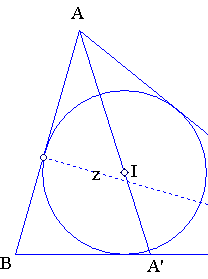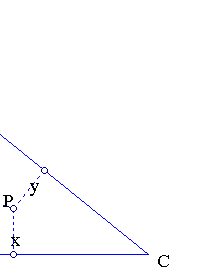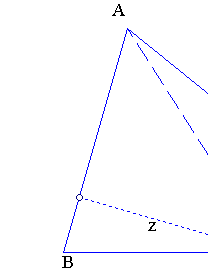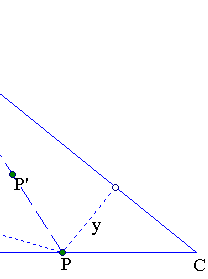Points on the sides of ABC have corresponding coordinate zero. For example, P on BC is characterized by trilinears of the form (0, y, z). These are directly related to the oriented ratio PB/PC = - (z/sinB)/(y/sinC) = -(z/y)*(sinC/sinB) = -(z/y)*(c/b) => z/y = -(PB/PC)*(b/c).

 Drawing a parallel to BC from P' we see that all points P' of line AP are characterized by the equation z/y = - k <==> z + k*y = 0, with k = (PB/PC)*(b/c). Thus, the median from A is characterized by (PB/PC = -1) z/y = b/c <==> b*y - c*z = 0. Analogously the bisector from A is characterized by (PB/PC = - c/b) z/y = 1 <==> z - y = 0.

 In general a line L is represented in this coordinate system by an equation of the form u*x + v*y + w*z = 0, {u,v,w} being defined modulo a non-zero multiplicative constant and being also not simultaneously zero.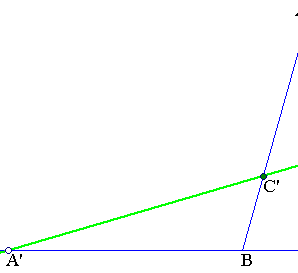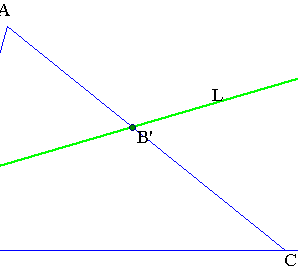The coefficients {u,v,w} are directly related to the coordinates of the intersection points of L with the sides of the triangle of reference: A'(0,y1,z1), B'(x2,0,z2), C'(x3,y3,0).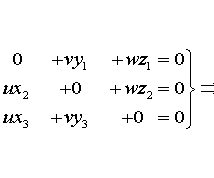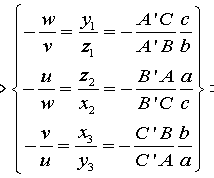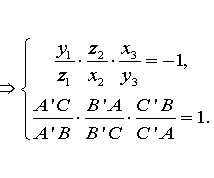The two last equations are equivalent to Menelaos theorem and each represents a necessary and sufficient condition for the existence of a line L passing through the three points A'(0,y1,z1), B'(x2,0,z2), C'(x3,y3,0) lying on the sides of triangle ABC.

The interpretation of the coordinates in terms of a model of the projective plane can be described as follows:

 In the three dimensional space R3, consider the plane (E) defined by the equation a*x+b*y+c*z = 2*D. Each line [x,y,z] of R3 through the origin, not parallel to (E) i.e. not satisfying a*x+b*y+c*z = 0, represents a point [X] of the projective plane and intersects (E) at a point PX = (x0,y0,z0) which are the absolute trilinears of PX.

 Points [X] = [x,y,z] with a*x+b*y+c*z = 0 represent the line at infinity. Adding these points to the ordinary points of (E) we obtain the projectification of plane (E).

 Each ordinary line L: u*x+v*y+w*z = 0 of (E) intersects the line at infinity a*x+b*y+c*z = 0 at the point (at infinity), whose coordinates are given by the vector product UxF = ((v*c-w*b), (w*a-u*c), (u*b-v*a)), where U=(u,v,w) and F=(a,b,c).

 This point represents the direction of line L and lines L', L'' , ... parallel to L define the same point at infinity S = (c1,c2,c3) satisfying a*x+b*y+c*z = 0. Hence the family of all lines parallel to that direction is defined through the solutions (in u,v,w) of the system: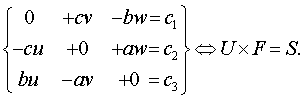The system is a compatible one of rank two, thus defining a one-parameter family of lines. The U's representing the solutions define a vector orthogonal to S, thus a linear combination of the form U = rF + t(FxS). Replacing in the above equation we find that r can be arbitrary and t = (F,F)-1.

 For a particular point P0=(x0,y0,z0) in (E) the line through that point must satisfy (U,P0) = ux0+vy0+zw0=0 ==> (U,P0) = r(F,P0) + t(FxS, P0)=0 ==> r = -(t/(2*D))*(FxS, P0). Thus, U is a non-zero multiple of [(2*D)*(FxS) - (FxS, P0)*F], P0 denoting absolute coordinates.

 In absolute trilinears the inner product (F,X) = 2*D, hence for these coordinates the equation of the line (U,X) = 0 becomes (FxS,X-X0) = 0.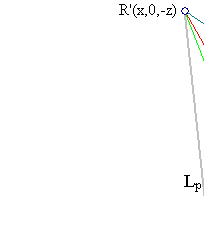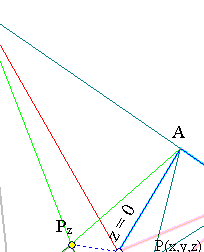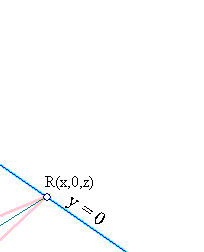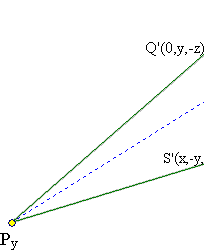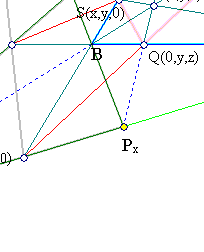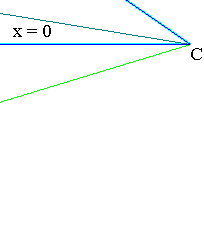The trilinears, as with any projective coordinate system, allow to write the points of the plane as linear combinations P = xA+yB+zC. This is handy when calculating cross ratios along lines, as for example with the points {Q,R,S} called traces of P on the sides of ABC and defined through the cevians of a point P. Q = yB+zC => Q' = yB - zC is the harmonic conjugate with respect to {B,C}. Analogously the other conjugate harmonics R', S'. The trilinears of {Q',R',S'} satisfy  and define the trilinear polar of P(x0,y0,z0), given by the equation: (y0*z0)*x+(x0*z0)*y+(x0*y0)*z=0 <==> (x/x0) + (y/y0) + (z/z0) = 0.

 In the same spirit we can write P = Q+xA and for the harmonic conjugate of P w.r. to A,Q: Px=Q-xA=(-x,y,z). Analogously we define the other harmonic associates Py, Pz of P with respect to the triangle.

 The above picture (to be encountered in many places, see for example in TriangleConics.html ), which Steve Sigur quotes as the most important picture in triangle geometry, suggests some collinearities. For example {C,Px,S',Py} are collinear and define a harmonic division. This is seen by writing: Px = P-(2x)A, Py = P-(2y)B ==> Px + Py = 2P-(2x)A-(2y)B = (2z)C, thus C is on the line of {Px, Py}. Analogously Px - Py = (2y)B - (2x)A = 2(yB-xA) = S' (since coordinates can be multiplied by a non-zero constant). This shows that S' is also on the line of {Px,Py} and since C = Px + Py and S' = Px - Py, the two points are harmonic conjugates w.r. to {Px, Py} (see ProjectiveBase.html ).

 It is also easily seen that line Lp is trilinear polar of P with respect to all three triangles ABC, PxPyPz and QRS.

 The selection of the incenter as the central point (or coordinator) of the projective coordinate system has some arbitrariness in it, but is useful in establishing properties of triangle centers. Any point of the plane of the triangle, not lying on the side-lines, could be considered as the coordinator and establish a new coordinate system of generalized trilinears. Another traditional choice, for example, is the centroid of the triangle, which defines the projective coordinate system of barycentric coordinates.

 Selecting two such coordinate systems {A,B,C,D} and {A',B',C',D'} where in each system D (resp. D') plays the role of coordinator (i.e. D = A+B+C, resp. D' = A'+B'+C'), the corresponding coordinates are related by a matrix relation of the form X' = MX, the matrix M being invertible and having columns the coordinates of {A,B,C} with respect to {A',B',C'}. In particular, systems with A=A', B=B', C=C' but different D, D' have a diagonal matrix, whose entries are the coordinates of D with respect to {A',B',C'}.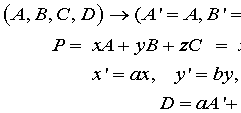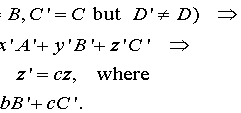For example, the relation between Barycentric (X=(x,y,z)) and standard trilinears (X'=(x',y',z')), as defined in , is
X' = (x/a, y/b, z/c), since (1/a, 1/b, 1/c) are the trilinears of the centroid.
More general, selecting coordinator D whose trilinears are (d1,d2,d3), the corresponding system (x,y,z) is related to the ordinary trilinears (X'=(x',y',z')) through X'=(d1*x, d2*y, d3*z) and naturally x = x'/d1, y = y'/d2, z = z'/d3.
A line with equation px+qy+rz=0, has in trilinears the equation (p/d1)x'+(q/d2)y'+(r/d3)z'=0.
A conic with equation pyz+qzx+rxy=0, has in trilinears the equation (pd1)y'z'+(qd2)z'x'+(rd3)x'y'=0.

 In particular, the line at infinity is easily calculated in barycentrics to have the equation x+y+z=0.
It follows, that in trilinears it has the equation ax'+by'+cz'=0, and in more general systems (see IsogonalGeneralized.html ) with coordinator D (=(d1,d2,d3) in the standard trilinears) it has the equation (a*d1)x + (b*d2)y + (c*d3)z = 0.
The circle, which in the system with coordinator D equal to the symmedian point has equation yz+zx+xy=0, in trilinears has the representation ay'z'+bz'x'+cx'y'=0 and a2yz+b2zx+c2xy=0 in barycentric coordinates.

BarycentricCoordinates.html
BarycentricsFormulas.html
Harmonic.html
IsogonalGeneralized.html
ProjectiveBase.html
ProjectiveCoordinates.html
TriangleConics.html
TrilinearPolar.html
TrilinearsRelatedToOthers.html
Tripole.html

### References

Sigur, S. Triangle Web Page http://paideiaschool.org/TeacherPages/Steve_Sigur/geometryIndex.htm
Yiu, P. GeometryNotes020402 (pdf). http://www.math.fau.edu/yiu/GeometryNotes020402.pdf